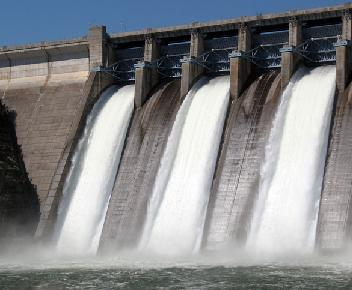# Calculation of Hydro Power

Before embarking on any hydro power generation project it is essential to survey the proposed site to calculate the amount of available hydro power.The two vital factors to consider are the flow and the head of the stream or river. The flow is the volume of water which can be captured and re-directed to turn the turbine generator, and the head is the distance the water will fall on its way to the generator. The larger the flow – i.e. the more water there is, and the higher the head – i.e. the higher the distance the water falls – the more energy is available for conversion to electricity. Double the flow and double the power, double the head and double the power again.

A low head site has a head of below 10 metres. In this case you need to have a good volume of water flow if you are to generate much electricity. A high head site has a head of above 20 metres. In this case you can get away with not having a large flow of water, because gravity will give what you have an energy boost.

The key equation to remember is the following:

Power = Head x Flow x Gravity

where power is measured in Watts, head in metres, flow in litres per second, and acceleration due to gravity in metres per second per second.
The acceleration due to gravity is approximately 9.81 metres per second per second – i.e. each second an object is falling, its speed increases by 9.81 metres per second (until it hits its terminal velocity).

Therefore it is very simple to calculate how much hydro power you can generate.
Let’s say for example that you have a flow of 20 litres per second with a head of 12 metres. Put those figures in the equation and you will see that:

12 x 20 x 9.81 = 2,354 Watts

## Automatic Hydro Power Calculator

Below is our automated hydro-power calculator. Simply enter the head in metres, and the flow rate in litres per second, and the calculator will work out the amount of power carried by the water and the amount of power which can realistically be extracted from it to generate electricity.

Head: (metres), Flow Rate: (litres per second)
Hydro Power: (Watts)
At 60% system efficiency (Watts) of generated electricity

### Real World Hydro Power Calculation

So in the example above a 12 metre head with a 20 litres per second flow rate equated to just over 2.3kW of available power. Sadly it is not possible to tap all of that power – nothing is 100% efficient. However, hydro power turbine generators are very efficient when compared to wind turbine generators and solar panels.

Efficiencies of around 70% can be expected which is to say that 70% of the hydraulic energy of the flowing water can be turned into mechanical energy spinning the turbine generator. The remaining 30% is lost. Energy is again lost in converting the mechanical energy into electrical energy (electricity) and so at the end of the day you can expect a complete system efficiency of around 50-60%.

In our previous example where 2.3kW of power was available – we can therefore expect to generate around 1.1-1.4kW of electricity.

These same calculations are valid whether you are planning a tiny Pico or Micro Hydro Power system or the next Three Gorges Dam Hydro Project.

Find out much more about hydro power by clicking here and viewing our Hydro Power Directory. We also have an Introduction to Hydro Electric Power, and information about the most common (small scale) Run of River Hydro Power systems. Waterwheels are introduced here.